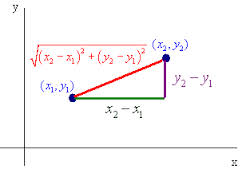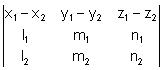Click to Chat

1800-1023-196

+91-120-4616500

CART 0

• 0

MY CART (5)

Use Coupon: CART20 and get 20% off on all online Study Material

ITEM
DETAILS
MRP
DISCOUNT
FINAL PRICE
Total Price: Rs.

There are no items in this cart.
Continue Shopping```Shortest Distance between Two Non-Intersecting Lines

Table of Content

Formula of Distance

Straight Line

Shortest Distance Between Two Non-Intersecting Lines

Related ResourcesBefore we proceed towards the shortest distance between two lines, we first try to find out the distance formula for two points.

Formula of Distance

If there are two points say A(x1, y1) and B(x2, y2), then the distance between these two points is given by √[(x1-x2)2 + (y1-y2)2].View the following video for more on distance formula:

Straight Line

A straight line in space is characterized by the intersection of two planes which are not parallel and hence the equation of straight line is in fact the solution of the system consisting of the equation of the planes:

a1x + b1y + c1z + d1 = 0 and a2x + b2y + c2z + d2 = 0

Such form of equation is also termed as the unsymmetrical form.

We now try to find the equation of the straight line in symmetrical form:

A(x1, y1, z1) be a given point on the straight line and l, m and n are the dc’s, then its equation is given by

(x-x1)/l = (y-y1)/m = (z-z1)/n = k (say)

Clearly, any general point on this line at a distance ‘k’ from the point A(x1, y1, z1) is given by P(x1 + lk, y1 + mk, z1 + nk).

Shortest Distance Between Two Non-Intersecting Lines

Now we discuss the condition for non-intersecting lines. Two lines are called non intersecting if they do not lie in the same plane. The straight line which is perpendicular to each of non-intersecting lines is called the line of shortest distance. And length of shortest distance line intercepted between two lines is called length of shortest distance. Let us discuss the method of finding this line of shortest distance.

Method: Let the equation of two non-intersecting lines be

(x–x1) / l1 = (y–y1) /m1 = (z–z1) /n1 = r1 (say)                               ……(1)

And (x–x2)/ l2 = (y–y2) /m2 = (z–z2) /n2 = r2 (say)                          ……(2)

Any point on line (1) is of the form P (x1 + l1r1, y1 + m1r1, z1 + n1r1)

and on line (2) is Q (x2 + l2r2, y2 + m2r2, z2 + n2r2).

Let PQ be the line of shortest distance. Its direction ratios will be

[(l1r1 + x1 – x2 – l2r2), (m1r1 + y1 – y2 – m2r2), (n1r1 + z1– z2 – n2r2)]

This line is perpendicular to both the given lines. By using the condition of perpendicularity we obtain 2 equations in r1 and r2.

And hence by solving these, values of r1 and r2 can be found. Subsequently he points P and Q can be found. The distance PQ is shortest distance.

The shortest distance can be found by PQ =Remark: If any straight line is given in general form then it can be transformed into symmetrical form and we can further proceed.

Illustration:

Find the shortest distance between the lines,

(x–3)/3 = (y –8)/–1 = (z–3)/1,

(x + 3)/–3 = (y+7)/2 = (z–6)/4.

Also find the equation of the line of shortest distance.

Solution:

Given lines are

(x – 3)/3 = (y – 8)/–1 = (z– 3)/1 = r1 (say)          ……(1)

(x + 3)/–3 = (y +7)/2 = (z – 6)/4 = r2 (say)          ……(2)

Any point on line (1) is of the form P (3r1 + 3, 8 – r1, r1 + 3)

and on line (2) is of the form Q (–3 – 3r2, 2r2 – 7, 4r2 + 6).

If PQ is line of shortest distance, then direction ratios of PQ

= (3r1 + 3) – (–3 – 3r2), (8 – r1) – (2r2 – 7), (r1+ 3) – (4r2 + 6)

i.e. 3r1 + 3r2 + 6, –r1 – 2r2 + 15, r1 – 4r2 – 3

As PQ is perpendicular to lines (1) and (2)

∴ 3(3r1 + 3r2 + 6) – 1(–r1 – 2r2 + 15) + 1(r1 – 4r2 - 3) = 0

⇒11r1 + 7r2 = 0                                              ……(3)

and –3(3r1 + 3r2 + 6) + 2(–r1 – 2r2 + 15) + 4(r1 – 4r2 - 3) = 0

i.e. 7r1 + 11r2 = 0                                              ……(4)

On solving equations (3) and (4), we get r1 = r2= 0.

So, point P = (3, 8, 3) and Q = (–3, –7, 6).

∴ Length of shortest distance PQ = √{(–3–3)2 + (–7–8)2 + (6–3)2} = 3√30

Direction ratios of shortest distance line are 2, 5, –1.

∴ Equation of shortest distance line is

x–3/2 = y–8/5 = z–3/–1.

Illustration:

Consider the lines

L1 = (x+1)/3 = (y+2)/1 = (z+1)/2

L2 = (x-2)/1 = (y+2)/2 = (z-3)/3

Find the unit vector perpendicular to both L1 and L2. Also find the shortest distance between the two. (2008)

Solution:

We may represent the given lines in vector form as

L1 = (-i-2j-k) + λ (3i+j+2k)

L2 = (2i-2j+3k) + μ (i+2j+3k)

Since, the vector is perpendicular to both L1 and L2 and so by solving with the help of determinants we obtain it as -i-7j+5k.

Hence, the required unit vector is (-i-7j+5k)/√[(-1)2 + (-7)2 + (5)2]

= 1/5√3. (-i-7j+5k)

The shortest distance between L1 and L2 is

|[(2-(-1))i + (2-2)j + (3-(-1))k] . (-i-7j+5k)/ 5√3|

= | (3i + 4k). (-i-7j+5k)/ 5√3|

= 17/ 5√3

Related Resources

Look into the past year papers to get an idea about the types of questions asked in the exam.

Various Recommended Books of Mathematics are just a click away.

To read more, Buy study materials of 3D Geometry comprising study notes, revision notes, video lectures, previous year solved questions etc. Also browse for more study materials on Mathematics here.
```### Course Features

• 731 Video Lectures
• Revision Notes
• Previous Year Papers
• Mind Map
• Study Planner
• NCERT Solutions
• Discussion Forum
• Test paper with Video Solution